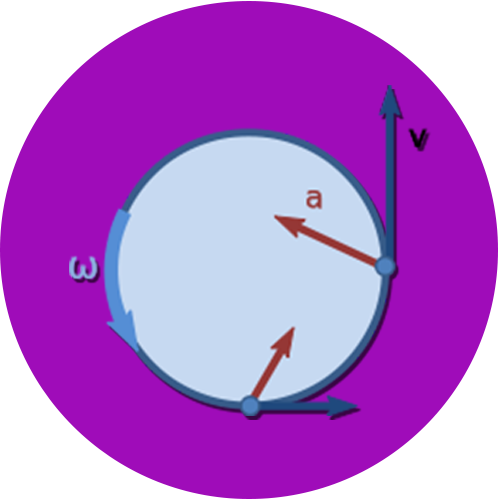# Circular Velocity Calculator

Use our circular velocity calculator a free online tool to calculate the circular velocity for the given inputs. Enter the values of radius and time period to calculate.

## Circular Velocity Formula

The circular velocity formula is given by:
v = 2πr/t
where,
v = circular velocity
r = Radius of the circular orbit
t = Time period
Pi (Π)= 3.14 as a constant used for the calculation.### What is Centripetal Velocity ?

In physics, the circular motion is define as the movement of an object along the circumference of a circle or rotation along its circular path. The object is said to move in uniform motion and has a constant angular rate of rotation and speed or non-uniform motion with changing rate of rotation. Objects moving in circles possess a speed which is equal to the distance travelled per time of travel. Thus, the speed of the object in circular motion is called circular velocity of that object.